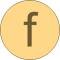# length

Return length of a vector (better as norm(), if further symbolic processing is performed)# Information

This information is part of the Modelica Standard Library maintained by the Modelica Association.

#### Syntax

```Vectors.length(v);
```

#### Description

The function call "`Vectors.length(v)`" returns the Euclidean length "`sqrt(v*v)`" of vector v. The function call is equivalent to Vectors.norm(v). The advantage of length(v) over norm(v)"is that function length(..) is implemented in one statement and therefore the function is usually automatically inlined. Further symbolic processing is therefore possible, which is not the case with function norm(..).

#### Example

```  v = {2, -4, -2, -1};
length(v);  // = 5
```